Transformations Question submitted by (15 January 2000)Return to The Archives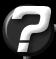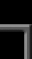I've noticed that some game engines, when doing rotations and transfomations, reset the identity matrix on each loop while others keep building on the original matrix ..is there any advantage of doing one over the other.... are some functions, such as collision detection easier to implement using one particular method...Thanks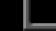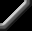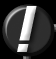There is a reason for going with each.First, if you use accumulated rotations (i.e. not resetting to identity each frame) then you can avoid the problems of gimble-lock (i.e. pitching more than 90 degrees in either direction.) However, you lose the advantage of absolute rotational values. In other words, you don't know your exact roll/pitch/yaw. Can you calculate roll/pitch/yaw from a matrix? Yes and no. The problem is that there can be more than one set of absolute rotational values for a given matrix. For example (try to follow this in your head) a pitch up of 90 degrees followed by a roll of 10 degrees could be the same as a yaw of 10 degrees followed by a pitch up of 90 degrees. So, what's the correct answer? They're both correct.Also, when you use cumulative rotations, you will also accumulate error over time. Your orthogonal rotation matrix could become skewed (i.e. no longer orthogonal.) Just for clarity, an orthogonal matrix is defined as a matrix whose three column- (or row-) vectors have a length of 1.0 and are all perpendicular to one another. As you accumulate rotations over and over, you'll accumulate error and will eventually end up with a really screwy matrix. Fortunately, there's a simple solution to this. For an example of how this is done, check out my handy-dandy multi-purpose matrix class (shameless plug :) at: ftp://ftp.fluidstudios.com/pub/FluidStudios/VectorMath/Fluid_Studios_Matrix_Template_Class.zipThen look for the routine called orthoNormalizeResponse provided by Paul Nettle